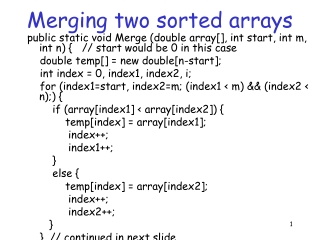DownloadDownload PresentationMerging two sorted arrays

# Merging two sorted arrays

Télécharger la présentation## Merging two sorted arrays

- - - - - - - - - - - - - - - - - - - - - - - - - - - E N D - - - - - - - - - - - - - - - - - - - - - - - - - - -
##### Presentation Transcript

1. Merging two sorted arrays public static void Merge (double array[], int start, int m, int n) { // start would be 0 in this case double temp[] = new double[n-start]; int index = 0, index1, index2, i; for (index1=start, index2=m; (index1 < m) && (index2 < n);) { if (array[index1] < array[index2]) { temp[index] = array[index1]; index++; index1++; } else { temp[index] = array[index2]; index++; index2++; } } // continued in next slide

2. Merging two sorted arrays for(;index1<m;index1++,index++) { temp[index] = array[index1]; } for(;index2<n;index2++,index++) { temp[index] = array[index2]; } for (i=start;i<n;i++) { array[i] = temp[i-start]; } }

3. Merge sort • Recursively sort half of the array separately and merge them class MergeSort { public static void main (String arg[]) { int n = 20; double array[] = new double[n]; Initialize (array, n); // not shown Sort (array, 0, n-1); } // continued in next slide

4. Merge sort public static void Sort (double array[], int start, int end) { if (start < end) { Sort (array, start, start+(end+1-start)/2-1); Sort (array, start+(end+1-start)/2, end); Merge (array, start, start+(end+1-start)/2, end+1); } } } // end class

5. Merge sort • Running time? Let it be T(n) for an array of size n T(n) = 2T(n/2) + cn for some constant c • Solution to this functional equation (or recurrence) is the running time of merge sort • T(n) = c’nlog2(n) for some constant c’ and large n • Note that this is the worst case running time of merge sort • Much better than bubble sort and insertion sort which had worst case running time quadratic in n

6. Use a static variable class StaticDemo { int x; // a normal instance variable static int y; // a static variable } class SDemo { public static void main(String args[]) { StaticDemo ob1 = new StaticDemo(); StaticDemo ob2 = new StaticDemo(); /* Each object has its own copy of an instance variable. */ ob1.x = 10; ob2.x = 20; System.out.println("Of course, ob1.x and ob2.x " + "are independent."); System.out.println("ob1.x: " + ob1.x + "\nob2.x: " + ob2.x); System.out.println();

7. Use a static variable /* Each object shares one copy of a static variable. */ System.out.println("The static variable y is shared."); ob1.y = 19; System.out.println("ob1.y: " + ob1.y + "\nob2.y: " + ob2.y); System.out.println(); System.out.println("The static variable y can be" + " accessed through its class."); StaticDemo.y = 11; // Can refer to y through class name System.out.println("StaticDemo.y: " + StaticDemo.y + "\nob1.y: " + ob1.y + "\nob2.y: " + ob2.y); } }

8. Use a static method class StaticMeth { static int val = 1024; // a static variable // a static method static int valDiv2() { return val/2; } } class SDemo2 { public static void main(String args[]) { System.out.println("val is " + StaticMeth.val); System.out.println("StaticMeth.valDiv2(): " + StaticMeth.valDiv2()); StaticMeth.val = 4; System.out.println("val is " + StaticMeth.val); System.out.println("StaticMeth.valDiv2(): " + StaticMeth.valDiv2()); } }

9. Static method • Can call only other static methods. • Must access only static data. • Do not have ‘this’ reference.

10. Use a static block class StaticBlock { static double rootOf2; static double rootOf3; static { System.out.println("Inside static block."); rootOf2 = Math.sqrt(2.0); rootOf3 = Math.sqrt(3.0); } StaticBlock(String msg) { System.out.println(msg); } } class SDemo3 { public static void main(String args[]) { //StaticBlock ob = new StaticBlock("Inside Constructor"); System.out.println("Square root of 2 is " + StaticBlock.rootOf2); System.out.println("Square root of 3 is " + StaticBlock.rootOf3); } }